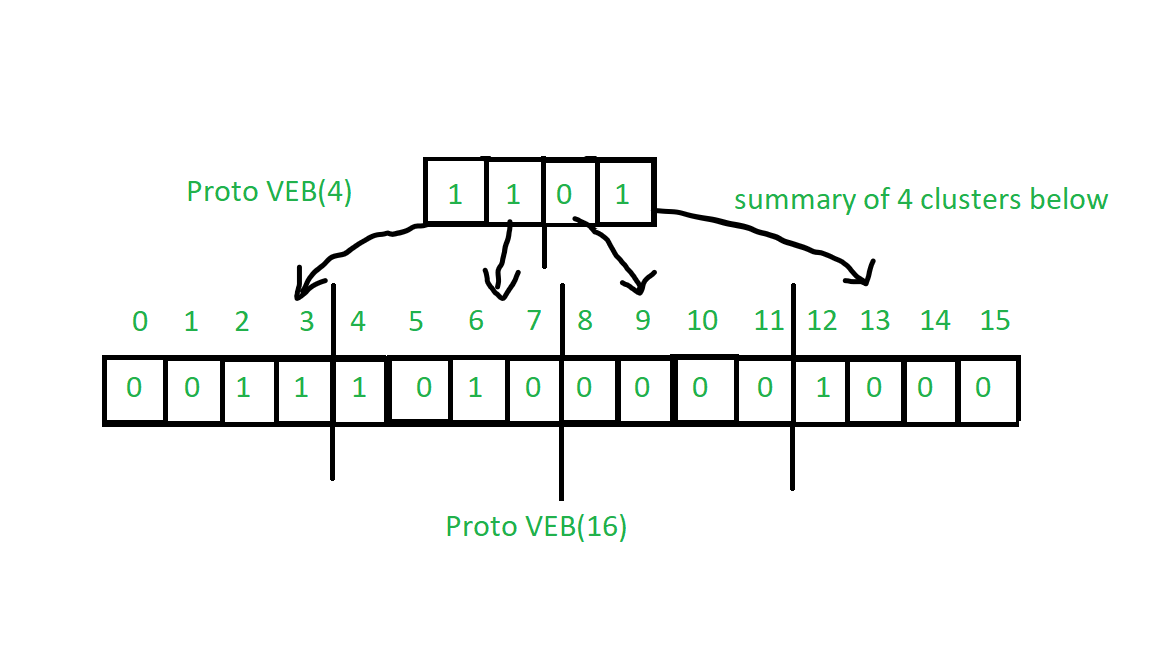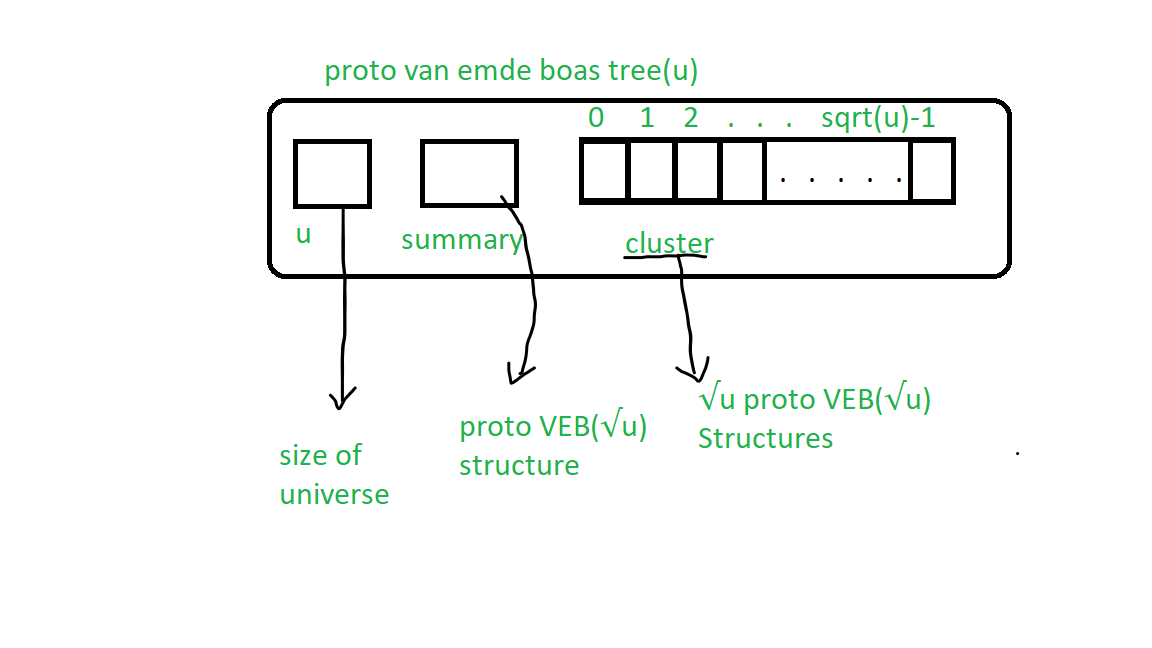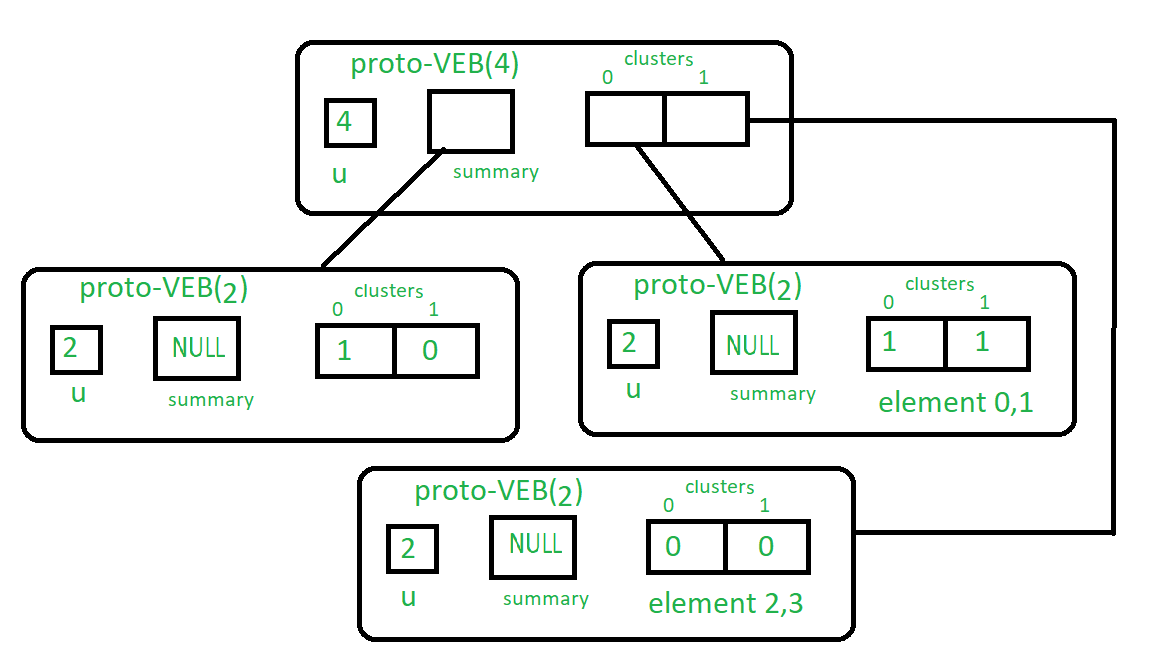GeeksforGeeks App
Open AppBrowser
Continue

# Proto Van Emde Boas Tree | Set 2 | Construction

Van Emde Boas Tree supports search, minimum, maximum, successor, predecessor, insert and delete operations in O(lglgN) time which is faster than any of related data structures like priority queue, binary search tree, etc. Proto Van Emde Boas tree is similar prototype type data structure but it fails to achieve the complexity of O(lglgN), We will learn Proto Van Emde Boas tree first to get a basic understanding of working of Van Emde Boas tree. Here N is the size of the universe over which tree is defined.

Note: Proto Van Emde Boas Data Structure’s keys must be defined over a range of 0 to n(n is positive integer of the form 22k) and it works when duplicate keys are not allowed.

Abbreviations:

1. Proto-VEB is an abbreviation of Proto-Van Emde Boas tree.
2. Proto-VEB() is an abbreviation for Proto-VEB containing u number of keys.

Basic Understanding of the structure of Proto-VEB Tree:
Proto Van Emde boas tree are recursively defined data structure and it shrinks to sqrt size as we go up in the tree. Please refer this article to understand the basics of it.

In Proto-VEB we use a bit array to represents whether a key is present or not, we put 1 if it is present and 0 else.
Here, the summary of a particular cluster contains whether there is any key present in a cluster if at least one key present then the summary is 1 or 0 elsewhere. Clusters are segments of a bit array. A summary is also a bit array. See the image below:Construction of Proto VEB Tree:
Below image represents the basic Proto-VEB structure:The recursively defined structure has two main part:

1. summary: it is a pointer to Proto-VEB structure which hassize.
2. cluster: it is an array of pointers to Proto-VEB structures withsize.

First of all, we have to understand some function and keywords:

• universe size (u) : Number of keys in Proto-VEB structure.
• High(x): From the first image, we can see that if we want to reach at the cluster of the key then we can divide it with.
For example, We want to know the cluster of the key 12 then can divide it withwhich is 3, so key 12 is in 3rd cluster.
High(x) = floor( x /)
• low(x): From the first image, we can see that if we want the position of the key in the cluster we can apply modulus operation x %.
For example, If you want to find a position of 7 in the cluster you can apply 7 %= 3 which is a position of 7 in 2nd cluster.
low(x) = x %)

Recursive Procedure of construction:

1. Base case : If universe size is 2 then it is a base size so there will be no more summary array, which means it is null and we will store only bit array for 2 keys.
2. We will recursively assign summary assized Proto-VEB tree and-sized Proto-VEB to allclusters.

See u=4 Proto-VEB structure in the image below:Here is the code representing the Algorithm:

## C++

 #include using namespace std; class Proto_Van_Emde_Boas {public:    // Total number of keys    int universe_size;     // Summary    Proto_Van_Emde_Boas* summary;     // Clusters array of Proto-VEB pointers    vector clusters;     int root(int u)    {        return (int)sqrt(u);    }     // Function to return cluster numbers    // in which key is present    int high(int x)    {        return x / root(universe_size);    }     // Function to return the position    // of x in cluster    int low(int x)    {        return x % root(universe_size);    }     // Function to return index form    // cluster number and position    int generate_index(int cluster, int position)    {        return cluster * root(universe_size) + position;    }     // Constructor    Proto_Van_Emde_Boas(int size)    {        universe_size = size;         // Base case        if (size <= 2) {             // Set summary to nullptr as there is no            // more summary for size 2            summary = nullptr;             // Vector of two pointers            // nullptr in starting            clusters = vector(size, nullptr);        }        else {             // Assigning Proto-VEB(sqrt(u)) to summary            summary = new Proto_Van_Emde_Boas(root(size));             // Creating array of Proto-VEB Tree pointers of size sqrt(u)            // first all nullptrs are going to assign            clusters = vector(root(size), nullptr);             // Assigning Proto-VEB(sqrt(u)) to all its clusters            for (int i = 0; i < root(size); i++) {                clusters[i] = new Proto_Van_Emde_Boas(root(size));            }        }    }}; // Driver codeint main(){    Proto_Van_Emde_Boas pveb(4);}

## Java

 import java.util.*; class Proto_Van_Emde_Boas {    // Total number of keys    int universe_size;    // Summary    Proto_Van_Emde_Boas summary;     // Clusters array of Proto-VEB pointers    ArrayList clusters;     int root(int u) { return (int)Math.sqrt(u); }     // Function to return cluster numbers    // in which key is present    int high(int x) { return x / root(universe_size); }     // Function to return the position    // of x in cluster    int low(int x) { return x % root(universe_size); }     // Function to return index form    // cluster number and position    int generate_index(int cluster, int position)    {        return cluster * root(universe_size) + position;    }     // Constructor    Proto_Van_Emde_Boas(int size)    {        universe_size = size;         // Base case        if (size <= 2) {            // Set summary to null as there is no            // more summary for size 2            summary = null;             // ArrayList of two pointers            // null in starting            clusters                = new ArrayList(size);            for (int i = 0; i < size; i++) {                clusters.add(null);            }        }        else {            // Assigning Proto-VEB(sqrt(u)) to summary            summary = new Proto_Van_Emde_Boas(root(size));             // Creating array of Proto-VEB Tree pointers of            // size sqrt(u) first all nulls are going to            // assign            clusters = new ArrayList(                root(size));            for (int i = 0; i < root(size); i++) {                clusters.add(null);            }             // Assigning Proto-VEB(sqrt(u)) to all its            // clusters            for (int i = 0; i < root(size); i++) {                clusters.set(                    i, new Proto_Van_Emde_Boas(root(size)));            }        }    }} // Driver codeclass Main {    public static void main(String[] args)    {        Proto_Van_Emde_Boas pveb            = new Proto_Van_Emde_Boas(4);    }}

## C#

 using System;using System.Collections.Generic; class Proto_Van_Emde_Boas{    // Total number of keys    public int universe_size;     // Summary    public Proto_Van_Emde_Boas summary;     // Clusters array of Proto-VEB pointers    public List clusters;     public Proto_Van_Emde_Boas(int size)    {        universe_size = size;         // Base case        if (size <= 2)        {            // Set summary to null as there is no more summary for size 2            summary = null;             // Vector of two pointers, null in starting            clusters = new List(size);            for (int i = 0; i < size; i++)            {                clusters.Add(null);            }        }        else        {            // Assigning Proto-VEB(sqrt(u)) to summary            summary = new Proto_Van_Emde_Boas((int)Math.Sqrt(size));             // Creating array of Proto-VEB Tree pointers of size sqrt(u)            // first all nulls are going to assign            clusters = new List((int)Math.Sqrt(size));            for (int i = 0; i < Math.Sqrt(size); i++)            {                clusters.Add(new Proto_Van_Emde_Boas((int)Math.Sqrt(size)));            }        }    }     public int root(int u)    {        return (int)Math.Sqrt(u);    }     // Function to return cluster numbers in which key is present    public int high(int x)    {        return x / root(universe_size);    }     // Function to return the position of x in cluster    public int low(int x)    {        return x % root(universe_size);    }     // Function to return index form cluster number and position    public int generate_index(int cluster, int position)    {        return cluster * root(universe_size) + position;    }} // Driver codeclass Program{    static void Main(string[] args)    {        Proto_Van_Emde_Boas pveb = new Proto_Van_Emde_Boas(4);    }}

## Python3

 from typing import List class Proto_Van_Emde_Boas:    def __init__(self, size: int):        self.universe_size = size         # Base case        if size <= 2:            # Set summary to None as there is no            # more summary for size 2            self.summary = None             # List of two pointers            # None in starting            self.clusters: List[Proto_Van_Emde_Boas] = [None] * size        else:            # Assigning Proto-VEB(sqrt(u)) to summary            self.summary = Proto_Van_Emde_Boas(self.root(size))             # Creating array of Proto-VEB Tree pointers of            # size sqrt(u) first all nulls are going to            # assign            self.clusters: List[Proto_Van_Emde_Boas] = [None] * self.root(size)             # Assigning Proto-VEB(sqrt(u)) to all its clusters            for i in range(self.root(size)):                self.clusters[i] = Proto_Van_Emde_Boas(self.root(size))     def root(self, u: int) -> int:        return int(u ** 0.5)     def high(self, x: int) -> int:        return x // self.root(self.universe_size)     def low(self, x: int) -> int:        return x % self.root(self.universe_size)     def generate_index(self, cluster: int, position: int) -> int:        return cluster * self.root(self.universe_size) + position  # Driver codeif __name__ == '__main__':    pveb = Proto_Van_Emde_Boas(4)

## Javascript

 class Proto_Van_Emde_Boas {  constructor(size) {    this.universe_size = size;    if (size <= 2) {      this.summary = null;      this.clusters = new Array(size).fill(null);    } else {      this.summary = new Proto_Van_Emde_Boas(this.root(size));      this.clusters = new Array(this.root(size)).fill(null).map(() => new Proto_Van_Emde_Boas(this.root(size)));    }  }   root(u) {    return Math.sqrt(u) | 0;  }   high(x) {    return (x / this.root(this.universe_size)) | 0;  }   low(x) {    return x % this.root(this.universe_size);  }   generate_index(cluster, position) {    return cluster * this.root(this.universe_size) + position;  }} let pveb = new Proto_Van_Emde_Boas(4);

Time Complexity : O(sqrt(n))

My Personal Notes arrow_drop_up